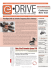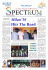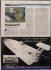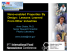# three dimensional magnetostatic analysis of a 3-phase, 12

## Transcription

three dimensional magnetostatic analysis of a 3-phase, 12
```THREE DIMENSIONAL MAGNETOSTATIC ANALYSIS OF A 3-PHASE,
12/8 POLE 250 W SWITCHED RELUCTANCE MOTOR USED IN A
WASHING MACHINE DRIVE
Ahmet FENERCİOĞLU1
1
Ünal KURT2
Gaziosmanpaşa University, Turhal Higher Vocational School, 60300 Turhal ,Tokat, Turkey, [email protected]
2
Amasya University, Amasya Higher Vocational School, 05189 Amasya, Turkey, [email protected]
Key Words: SRM design, finite element analysis, SRM magnetic analysis.
ABSTRACT
II. MATERIAL AND METHOD
In this study, physical sizes and geometry of a 3-phase,
12/8 pole, 250 watts switched reluctance motor (SRM)
which is used in a washing machine drive have been
determined and its one-to-one model has been
developed. The model obtained has been analyzed
through the 3 D magnetostatic analysis method and
3 D finite elements method. As a result of the analyze,
phase inductance, torque ripple, average and instant
torque, air – gap power and magnetic flux pattern
values have been obtained. Besides, analytical
approach values for these magnitudes have been
calculated and simulated. The fact that the results of
the analytical approaches and simulation results are
close to the real size values proved that the applied
simulation method was correct.
In this study, magnetostatic analysis of a three-phase,
12/8 SRM used in the washing machine drive through the
computer aided three dimensional finite elements method
by determining its real sizes and geometry. As a result of
the simulations, inductance, torque and power values
have been estimated and compared with the analytic
results [1–3].
I. INTRODUCTION
The theory of motor with variable reluctance has been
started to be used since 1980’s for the variable or adjusted
speed application and the use of these motors started to be
very popular in engineering applications. The advantages
brought by the cheap and powerful switching elements
enabled the re-discovery of this motor. SRM is very
simple in structure. However its control is complex. For
instance, the process of excitation of the phase windings
in appropriate order for torque generation requires the
provision of the position information of the rotor.
Besides, SRM’s have been enabled to compete with the
other DA and AA motors by the possibility of obtaining
low cost microprocessor and power electronic elements
[1, 2].
The values of physical changes of SRM with 12/8 pole
 are seen the Table 1. The real picture, simulator cross
section and size variables and values  are given in
Figure 1.
Table 1. Sizes of the SR Motor studied
Symbol
Meaning and Unit
Value
Rso
Rsi
Rro
Rri
Rsf
ly
lg
Lstk
Stator outer diameter (m)
Stator inner diameter (m)
Rotor outer diameter (m)
Rotor outer diameter (m)
Shaft diameter (m)
Stator yoke (m)
Air gap (m)
Stack length (m)
Stator pole number
Rotor pole number
Number of phase
Number of winding for a pole
0,06975
0,05725
0,04144
0,03050
0,00842
0,01250
0,00046
0,04630
0,2616
0,2704
12
8
3
200
βs
βr
ns
nr
q
N
(1). In Figure 2 the equivalent value for one phase of
SRM is shown [3,5].
Figure 2. Equivalent circuit for one phase of SRM
It is expressed as follows:
(a)
V = Ri +
dψ (θ , i )
di'
− M (θ )
dt
dt
(1)
Here the V is the supply voltage, R is the winding
resistance, ψ is the magnetic flux, i is the phase winding
current, M(θ) is the mutual inductance, θ is the rotor
position angle, i’ is the winding current excited before the
relevant phase. When saturation is neglected magnetic
flux is ψ = L(θ ,i) and there for Eq. (1) is re arranged as
follows:
V = Ri +
(b)
dL(θ )
di '
− M (θ )
dt
dt
(2)
Here L(θ ,i) shows the phase inductance. When the
differential expression in Eq. (2) is divided into pieces, it
becomes as follows by neglecting the mutual inductance;
V = Ri + L(θ )
di
dL (θ )
+i
dt
dt
(3)
When the mutual inductance value is neglected it
becomes as Eq. (3) and Eq. (4) [3,5].
dL(θ , i) dL(θ , i)
=
ω
dt
dθ
(4)
Here we obtain:
di
dL(θ )
di di ,
ω +i
ω
=
ω V = Ri + L(θ )
dθ
dθ
dt dθ
Here
(c)
Figure 1. Model of the 12/8 SR Motor which is studied.
a) Simulator model, b) Real model c) Size variables.
EQUIVALENT CIRCUIT OF SRM
Motor phase inductance in SRMs varies according to
rotor position and the current passing through the
winding. General circuit equation of SRM is given in Eq.
ω
(5)
is the angular velocity. [3,5]
In order to find the phase inductance in the intermediary
rotor positions of a SRM, cosine correlations are given in
Eq. 6 as a Fourier Series by using the maximum and
minimum inductance values.
m
L(θ , i ) = ∑ Lk (i) cos(knRθ )
k =0
(6)
Here L indicates the phase inductance (H), θ indicates
the rotor position in degrees, nr indicates the pole
number.
Here
ARM has low energy ratio. To express this, an energy
conversion cycle has been calculated magnetically. SRM
is a linear model in the first harmonic of the Fourier
Series of the inductance according to the SRM rotor
position. Here the inductance value is given in Eq. 7, flux
linkage is given in Eq. 8 [3, 6].
Magnetic devices are traditionally designed by combining
the simplified circuit models and rules based on
experimental evidence. However, since the devices are
increasingly variant and complex and the rules are not
sufficient, electromagnetic field problems have been
started to be solved according to the analysis and design
based on logical, detailed solution [1,3].
L(θ , i) = [(a0 − a1 cos(nRθ )]
(7)
λ (θ , i ) = [(a0 − a1 cos(nRθ )]i
(8)
Here λ is the flux linkage (wb), i is the phase current.
The a0 and a1 coefficients in Eq. 3 can be obtained from
Eq. 9 .
a0 =
1
1
( La + Lu ) , a1 = ( La − Lu )
2
2
(9)
Here nR is the number of rotor, for one phase, La is the
maximum inductance, Lu is the minimum inductance.
In the application, this motor is not run in the unaligned
position. The operation area of the motor is between the
overlap position and the aligned position of the rotor and
stator pole. After this position the same region is valid for
the 2nd phase and the excitation is cut and the 2nd phase
is excited when the 1st phase overlaps.
βs
is the angle of stator pole angle in degrees
III. RESULTS AND DISCUSSION
Phase inductances and instantaneous torque values of the
SRM model developed are estimated according to the
position change for each 2.5 degree of the rotor after the
magnetostatic analysis is made with the 3-dimensional
simulator. Besides these results are also obtained
analytically and compared in the graphic in Figure 3.
Here the rotor position is given between 0-45º. 0º-22,5º
indicates the generator operation region and 22,5º-45º
indicates the motor operation region. In both areas, the
concerned phase is not operated between 15º-30º which is
the unaligned region. In this region other phase is excited.
When the align of the stator pole of the phase with the
rotor pole finishes, the excitation of that phase is stopped
and the energy is supplied to the other phase. That is,
when the rotor pole starts overlapping with the stator,
phase excitation is started.
When there is a current pass a phase, the torque tends to
rotate the rotor in a direction to increase the inductance.
The direction of the torque is always towards the closest
overlapping position. Therefore positive torque (for
instance motor operation torque) can be generated only if
the rotor is between the non-overlapping forward position
and the next overlapping position. The most general
expression of the instantaneous torque caused by a phase
in each rotor position is given in Eq. 10 [3,4,6,7].
∂ i
  ∂W ' 
T =  ∫ λ (θ , i) di  = 

 ∂θ 0
  ∂θ  i = sabit
(10)
Here T is the instantaneous torque (Nm), W is the coenergy (J). Since the instantaneous torque changes
according to the rotor position, mean torque is a more
appropriate term to express the torque of a motor.
Considering the linear magnetic characteristic of SRM,
mean torque or electromagnetic torque is given in Eq. 11.
1 dL 1 2 La − Lu 1 2 La  Lu
1 −
Te = i 2
= i
= i
2 dθ 2
βs
2 β s  La



(11)
Figure 3. Change of phase inductance according to the
rotor position
The characteristic of the flux linkage between the aligned
and unaligned positions is given in Figure 4 (a) [3,4,7].
In figure 6, torque ripple of SRM motor is shown. Here,
torque ripple caused by the 45º movement of the rotor as
a result of consecutive triggering of 3 phases. But the
speed and inertia of the rotor has not been taken into
consideration, and only the static conditions are
considered. The speed, mass and inertia of the motor and
the control strategy of its drive circuit will further reduce
the torque ripple . In figure 6, torque ripple is given for
the 1/8 lap of the rotor according to the magnetostatic
simulation results.
(a)
(b)
Figure 4. a) Flux Linkage characteristics of SRM
b) flux distribution of aligned position
Figure 4 (b) shows the flux distribution of SRM in
aligned position as vectorial and magnitude values. In the
aligned position the local saturation in the pole corners is
at the minimum levels according to the flux distribution.
In positions where the rotor pole overlaps less with the
stator pole, this saturation effect is more. The material
used in the simulator model is the one called steel 1010
and the BH characteristics are given in figure 5. The knee
point of this material is around 1.9 T and saturation starts
after this value.
(b)
Figure 6. Torque ripple of SRM
In this case, the analytically estimated torque expression
and the values obtained by the 3 dimensional simulators
are given in Table 2 in comparison.
Table 2. Mean torque and power values
Solution
method
3B
simulator
Analytic
Mean
torque
(Nm)
Power (W)
(for 1000 d/d)
Motor Power
(W)
(Label Value)
250
2,377
2,558
248,78
267,78
250
Simulator results in Table 2 are predicted after the 3
dimensional magnetostatic analysis. The dynamic
operating conditions of the motor, its control strategy and
the effects of the drive circuit have not been taken into
consideration. Stator and rotor have been taken as a
whole in the motor design, and no lamination has been
made. Windings have been drawn as single-coiled and
each winding has been excited with 500 amper-tur mmk.
It is assumed that 2,5 A flow passes through a coil with
200 number of windings. The magnetic material used in
the simulation is not the same with the laminated material
in the real motor. BH curve is the material called steel
1010 in the 3D FEA simulator software library which is
a suitable material for SRM given in Figure 5.
Figure 5. B-H curve of the material (steel 1010) used as
rotor and stator core
The analytic results have been calculated according to the
equations given in the material and method section
according to the linear operating conditions. The torque
estimated through simulation and analytic method is the
electromagnetic torque as it is calculated according to the
co-energy. Therefore the power values estimated in Table
2 are the air gap power and the losses have been
neglected.
IV. CONCLUSION
It is more difficult to estimate the electrical and magnetic
characteristics of SRM than the other methods. Because,
inductance, power and energy amounts vary according to
the rotor position in SRM. SRM doesn’t have the stable
condition where all state variables are fixed. The
parameters changing according to the rotor position, local
magnetic saturation effects and variant magnetic
permeability affect the characteristics of SRM and
therefore the behaviour of SRM is attempted to be
increased through the simulation works rather than the
analytical methods.
The basic electrical parameters of the 3 phase, 12/8 pole
SRM which is examined in this study have been predicted
by making magnetostatic analysis through the three
dimensional finite elements method as well as the
analytic solutions. The results obtained are the analytic
estimates, simulation results and motor label values and it
is seen that these values are close when compared to each
other. In this case, the results provided by the study
proves the correctness of the simulation method, because
in order to conclude a correct design and analysis it is
necessary that the computer based simulation methods
for everything except basic sizing calculations are a part
of the design process. In particular, it is strongly
recommended to use the three dimensional simulators in
magnetic system design as the machine geometry cannot
be fully defined in two dimensional simulators and effects
like coil end-fields, axial flux, fringing flux and skewing
cannot be taken into account [3,9].
REFERENCES
1. Gürdal, O., “Elektrik Makinalarının Tasarımı”, Atlas
Yayın Dağıtım, İstanbul, 201-240, 2001.
2. Krishnan, R., “Switched Reluctance Motor Drives”,
CRC Press, Florida, 1-169, 2001.
3. Fenercioğlu, A., “Anahtarlamalı Relüktans Motorun
(HY-ARM) Tasarımı ve Analizi”, Doktora Tezi,
Gazi Üniversitesi Fen Bilimleri Enstitüsü, Ankara
2006.
4. Tarımer, İ., “Redüktörsüz Mekanik Sistemler İçin
Düşük Devirli Ve Yüksek Torklu Elektrik Motor
Tasarımları“, Doktora Tezi, Gazi Üniversitesi Fen
Bilimleri Enstitüsü, Ankara 2003.
5. Dursun, M., Saygın, A., “Güneş Enerjisi İle Çalışan
Bir Sulama Sistemi İçin Boost Konvertörlü
Anahtarlamalı Relüktans Motor Sürücüsü”, Erciyes
Üniversitesi Fen Bilimleri Ens. Dergisi, 22(1-2) 5765, 2006.
6. Gürdal,O., Fenercioglu, A., “Computer Aided Three
Dimensional Magnetostatic Analysis of Helically
Structured Switched Reluctance Motor (HS-SRM)”,
Journal of The Faculty of Engineering and
Architecture of Gazi University, 2007.
7. Omaç, Z., Kürüm, H., “Anahtarlamalı Reluktans
Motorun TMS320LF2407A Sayısal Sinyal İşlemcisi
ile PWM Akım Kontrolü”, Elektrik-Elektronik ve
Bilgisayar Mühendisliği Sempozyumu, Bursa 111116, 2004.
8. Şahin, F., Ertan, H.B., Leblebicioğlu, K., “Optimum
Geometry for Torque Ripple Minimization of
Switched Reluctance Motors”, IEEE Transactions
on Energy Conversion, 15(1):30-39, 2000.
9. Fenercioglu, A., Tarımer, "Anahtarlamalı Relüktans
Motorlarda Faz Endüktansına Etki Eden Faktörlerin
İncelenmesi", Pamukkale Üniversitesi, Mühendislik
Bilimleri Dergisi, 13.cilt, 2.sayı, 2007.
```

### frs_brz_sheet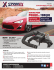### magureanu mihaela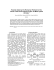### report on toyota/prius motor design and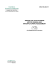### Granulation and automation technology for the plastics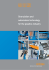### 4050 What is SRM? Broch5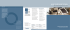### Datasheet Elotest M3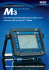### Bell Huey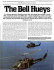### Granulation and automation technology for the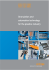### ASSESSMENT OF THE THREE-PHASE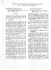### Thesis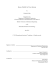### SLOT-LESS TORUS SOLID-ROTOR-RINGED LINE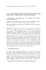### Presentation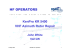### a new design method for low-speed torus type afpm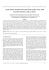### ETP Techno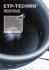### Honda 300ex National Race Machine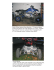### catalogue pages RUFLEX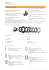### SensorlessIndirectStatorFieldOrientation Speed ControlforSingle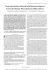### Experiments with Spiral Magnetic Motors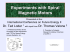### Winter - Service Wire